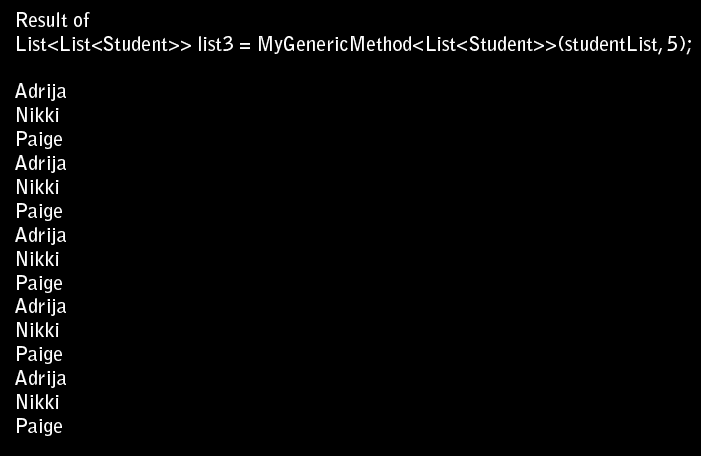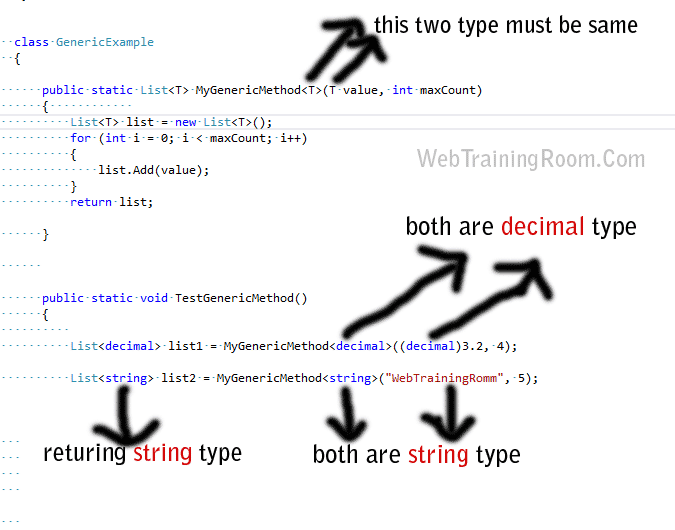# C# Generic Examples

What is generic in c#? C# generics is the concept of type parameters to .NET, which allow us to write generic method that can accept any type of object as input parameter and also can specify the type for output parameter, here we learn how to use Generic methods with some real-time examples.

Generics is the mechanism that allow us to define any specification, that can work with any data type of base class, not just any particular type, thus it increase the reusability.

Generic comes under `System.Collections.Generic` namespace.

## How to use Generic in C#

To understand Generic completely, we need to understand following usage of Generic

• Generic Type Parameters
• Generic Classes
• Generic Interfaces
• Generic Methods
• Generic Delegates

Here we learn c# generic method, First we create then we call the generics method

```// this is just normal class to be used in generic method
public class Student
{
public int StudentId { get; set; }
public string Fullname { get; set; }
public DateTime DOB { get; set; }
}```
c# generics method example

Here is an example of generic method name and generic return type

```class GenericExample
{
public static List<T> MyGenericMethod<T>(T value, int maxCount)
{ List<T> list = new List<T>();
for (int i = 0; i < maxCount; i++)
{
}
return list;

}
}```

Now we see how to call the generic method with generic values in C#
Here we have given three example of calling generic method with differnt type of values

```public static void TestGenericMethod()
{
//Calling Example 1
List<decimal> list1 = MyGenericMethod<decimal>((decimal)3.2, 4);
//Calling Example 2
List<string> list2 = MyGenericMethod<string>("WebTrainingRomm", 5);
//Calling Example 3
List<Student> studentList = new List<Student>();
studentList.Add(new Student() { Fullname = "Nikki", StudentId = 11, DOB = DateTime.Now });
studentList.Add(new Student() { Fullname = "Paige", StudentId = 12, DOB = DateTime.Now });
List<List<Student>> list3 = MyGenericMethod<List<Student>>(studentList, 5);
foreach (List<Student> s in list3)
{
foreach (Student stu in s)
{
Console.WriteLine(stu.Fullname);
}
}
}```

If you notice in above code, first we called the generic method with decimal type, then with string type and last call with a custom object type (“Student”)

Here is the output of last callJust remember in generic method what type of data you send as T vale, take a look at the picture belowMultiple interfaces can be specified as constraints on a single type

```class People<t> where T : System.IComparable<t>, IEnumerable<t>
{
}
```